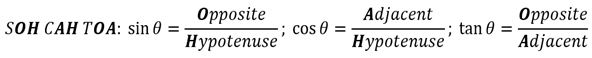# Find the exact value of trigonometric ratios

### Find the exact value of trigonometric ratios

#### Lessons• 1.
Find the exact value of all six trigonometric ratios, then make a conclusion
which primary trigonometric ratios (sin $\theta , cos \theta, tan \theta$) are positive in each
quadrant
a)
$\theta$=30°

b)
$\theta$=120°

c)
$\theta$=225°

d)
$\theta$=300°